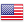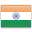Grade "A+" Accredited by NAAC with a CGPA of 3.46
Grade "A+" Accredited by NAAC with a CGPA of 3.46

# Advanced Financial Econometrics

Course ID
FD 504
Level
Program
BBA (FIA)
Semester
Fifth
Credits
6.0
Paper Type
Discipline Specific Elective
Method
Lecture & Tutorial

## Unique Paper Code: Update Awaited

This course provides a comprehensive introduction to financial econometric concepts and techniques. It covers financial time Series econometrics, regression models with cross- sectional financial data, Asset price volatility models, simultaneous-equation models in financial time series, and economic forecasting.

## Learning Outcomes:

At the end of the course, students should be able to:

• Understanding of financial econometric concepts and techniques
• Interpretation of regression models with cross-sectional financial data
• Understanding of Asset price volatility models
• Understanding of simultaneous-equation models in financial time series, and economic forecasting.

## Course Contents

Unit I
Unit II
Unit III
Unit IV

Unit I (3 weeks)

Financial time Series econometrics: Stationary stochastic processes, nonstationary Stochastic Processes, unit root stochastic processes, trend Stationary and difference Stationary stochastic processes. Tests of stationarity- graphical analysis and autocorrelation function (ACF) and correlogram statistical significance of autocorrelation coefficients. The unit root test – the augmented dickey-fuller (ADF) test. Transforming nonstationary financial time series – difference stationary processes and trend-Stationary process. Cointegration: regression of a unit root financial time series on another unit root financial time series, testing for cointegration and Cointegration and Error Correction Mechanism (ECM).

References:

Gujarati, N. Damodar. Basic Econometrics. New Delhi: McGraw Hill. [Chapter 21and 22]

Gujarati, N. Damodar. Econometrics by Examples. New Delhi: McGraw Hill.[Chapter 13 and 14]

Unit II (3 weeks)

Regression models with cross-sectional financial data: The logit and Probit models, multinomial regression models, Ordinal regression models, and Limited dependent variable regression models.

References:

Gujarati, N. Damodar. Basic Econometrics. New Delhi: McGraw Hill. [Chapter 15-17].

Gujarati, N. Damodar. Econometrics by Examples. New Delhi: McGraw Hill.[Chapter 8-11]

Unit III (4 weeks)

Asset price volatility: The ARCH and GARCH models. Extensions of the ARCH model. Simultaneous-equation models in financial time series: The nature of simultaneous-equation models, simultaneous-equation models, simultaneous-equation bias, inconsistency of OLS estimators. A test of simultaneity, tests for exogeneity. Simultaneous-Equation Methods – approaches to estimation, recursive models and ordinary least squares, estimation of a just identified equation, the method of indirect least squares (ILS), estimation of an overidentified equation: the method of two-stage least squares (2SLS)

References:

Gujarati, N. Damodar. Basic Econometrics. New Delhi: McGraw Hill. [ Chapter 17 – 20]

Gujarati, N. Damodar. Econometrics by Examples. New Delhi: McGraw Hill. [13 and 15]

Unit IV (2 weeks)

Economic forecasting: Forecasting with regression models. The Box–Jenkins methodology: ARIMA modeling. An ARMA model of companies daily closing prices. Vector autoregression (VAR), Testing causality using VAR: The Granger causality test

References:

Gujarati, N. Damodar. Basic Econometrics. New Delhi: McGraw Hill. [Chapter 22]

Gujarati, N. Damodar. Econometrics by Examples. New Delhi: McGraw Hill. [Chapter 16]

Recommendation Computer Package to be Used: Use of software like E Views, R and STATA solving real life problems.

#### Text Books

Christopher Dougherty. Introductory Econometrics. Oxford University Press.
Gujarati, N. Damodar. Basic Econometrics. New Delhi: McGraw Hill.
Gujarati, N. Damodar. Econometrics by Examples. New Delhi: McGraw Hill.

Pindyck, Robert S. and Daniel L. Rubinfeld Econometric Models and Economic Forecasts. Singapore: McGraw Hill.
Ramanathan, Ramu (2002). Introductory Econometrics with Applications (5th ed.). Thomson South Western

#### Teaching Learning Process

Class room lecture, Case study discussion, Numerical Problem solving, Class presentation on the assigned topic by students individually or in group, Workshop, Tutorials, Role play

#### Assessment Methods

1. Internal evaluation of 25% marks
a. Attendance 5% marks
b. Two internal evaluations by the teacher with 10% marks each out of which one must be a class test and other may be another test or home assignment or presentation. Faculty may take more than two assignments and (or) tests but total will be only 20% marks.
2. End term University Exam of 75% marks

#### Keywords

Autocorrelation, Error Correction Mechanism, ARCH, GARCH, OLS, Vector auto-regression,

Disclaimer: Details on this page are subject to change as per University of Delhi guidelines. For latest update in this regard please refer to the University of Delhi website here.Englishहिन्दी